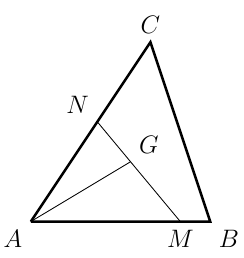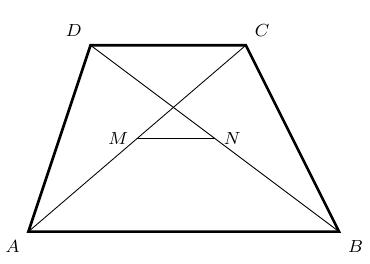###### back to index | new

Quadrilateral $APBQ$ is inscribed in circle $\omega$ with $angle P = \angle Q = 90^{\circ}$ and $AP = AQ < BP$. Let $X$ be a variable point on segment $\overline{PQ}$. Line $AX$ meets $\omega$ again at $S$ (other than $A$). Point $T$ lies on arc $AQB$ of $\omega$ such that $\overline{XT}$ is perpendicular to $\overline{AX}$. Let $M$ denote the midpoint of chord $\overline{ST}$. As $X$ varies on segment $\overline{PQ}$, show that $M$ moves along a circle.

Let unit vectors $a$, $b$, and $c$ satisfy $a+b+c=0$, prove the angles between these vectors are all $120^\circ$.

Let $G$ be the centroid of $\triangle{ABC}$. Points $M$ and $N$ are on side $AB$ and $AC$, respectively such that $\overline{AM} = m\cdot\overline{AB}$ and $\overline{AN} = n\cdot\overline{AC}$ where $m$ and $n$ are two positive real numbers. Find the minimal value of $mn$.Let $\triangle{ABC}$ be an acute triangle and $a, b, c$ be the three sides opposite to $\angle{A}, \angle{B}, \angle{C}$ respectively. If vectors $m=(a+c,b)$ and $n=(a-c, b-a)$ satisfy $m\cdot n = 0$, (1) Compute the measurement of $\angle{C}$. (2) Find the range of $\sin{A} + \sin{B}$.

Starting at the origin, a bug crawls 1 unit up, 2 units right, 3 units down and 4 units left. From this new point, the bug repeats this entire sequence of four moves 2015 more times, for a total of 2016 times. The coordinates of the bug’s final location are $(a, b)$. What is the value of $a + b$?

Let $ABCD$ be a trapezoid. Points $M$ and $N$ are the mid points of its diagonal $AC$ and $BD$, respectively. Show that $MN \parallel AB$ and $MN = \frac{1}{2}\mid AB - CD\mid$.The points $(0,0)$, $(a,11)$, and $(b,37)$ are the vertices of an equilateral triangle. Find the value of $ab$.